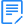# 会计日常工作中常用的Excel公式你掌握了多少？

1、文本与百分比连接公式

2、账龄分析公式

3、屏蔽错误值公式

4、完成率公式

5、同比增长率公式

6、金额大小写公式

7、多条件判断公式

8、单条件查找公式

9、双向查找公式

10、多条件查找公式

11、单条件求和公式

12、多条件求和公式

13、隔列求和公式

14、多表求和公式

15、两条查找相同公式

16、两表数据多条件核对

17、个税计算

18、会计科目截取公式

19、多表同一位置汇总公式

20、中国式排名公式

21、库存先进先出公式

1、文本与百分比连接公式

="本月利润完成率为"&TEXT(C2/B2,"0%")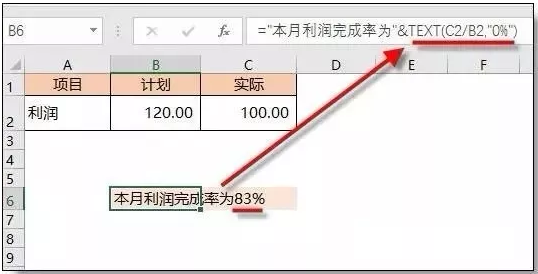2、账龄分析公式

=LOOKUP(D2,G\$2:H\$6)

=LOOKUP(D2,{0,"小于30天";31,"1~3个月";91,"3~6个月";181,"6-1年";361,"大于1年"})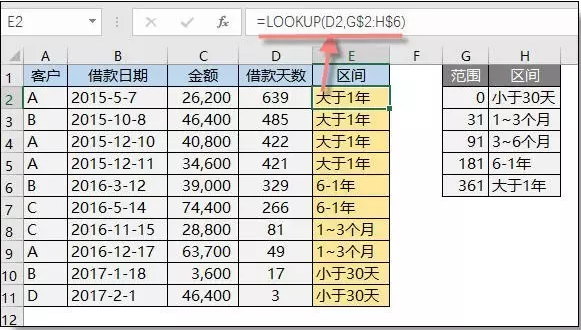3、屏蔽错误值公式

=IFERROR(A2/B2,"")4、完成率公式

=IF(C3<0,2-B3/C3,B3/C3)5、同比增长率公式

=(B2-C2)/IF(C2>0,C2,-C2)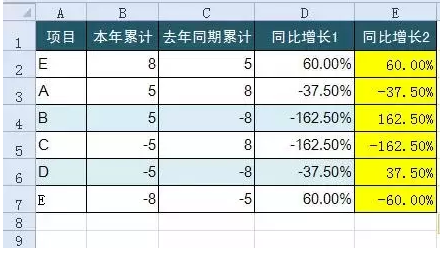6、金额大小写公式

=TEXT(LEFT(RMB(A2),LEN(RMB(A2))-3),"[>0][dbnum2]G/通用格式元;[<0]负[dbnum2]G/通用格式元;;")&TEXT(RIGHT(RMB(A2),2),"[dbnum2]0角0分;;整")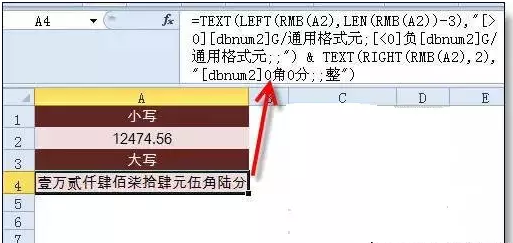7、多条件判断公式

=IF(AND(A2<500,B2="未到期"),"补款","")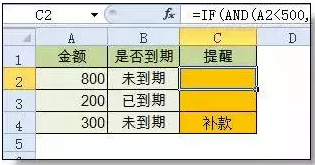8、单条件查找公式

=VLOOKUP(B11,B3:F7,4,FALSE)9、双向查找公式

=INDEX(C3:H7,MATCH(B10,B3:B7,0),MATCH(C10,C2:H2,0))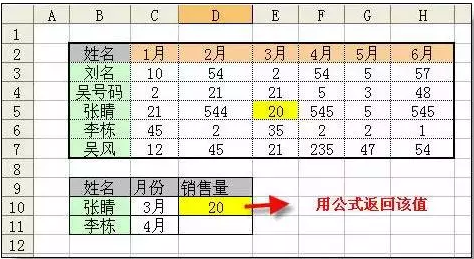10、多条件查找公式

=Lookup(1,0/((B25:B30=C33)*(C25:C30=C34)),D25:D30)11、单条件求和公式

=SUMIF(A:A,E2,C:C)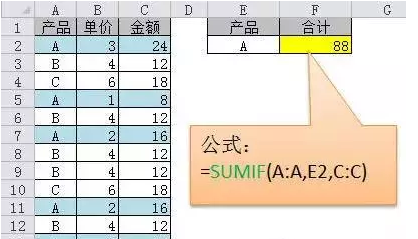12、多条件求和公式

=Sumifs(c2:c7,a2:a7,a11,b2:b7,b11)13、隔列求和公式

=SUMIF(\$A\$2:\$G\$2,H\$2,A3:G3)

=SUMPRODUCT((MOD(COLUMN(B3:G3),2)=0)*B3:G3)14、多表相同位置求和

=SUM(Sheet1:Sheet19!B2)15、两条查找相同公式

=COUNTIF(Sheet15!A:A,A2)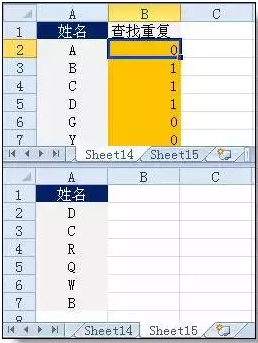16、两表数据多条件核对

=SUMPRODUCT((\$A\$2:\$A\$6=A10)*(\$B\$2:\$B\$6=B10)*\$C\$2:\$C\$6)-C10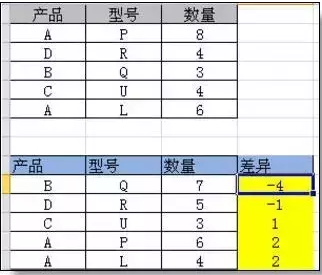17、个税计算

=5*MAX(A2*{0.6,2,4,5,6,7,9}%-{21,91,251,376,761,1346,3016},)

18、会计科目截取公式

=LEFT(B3,FIND("-",B3)-2)

=MID(B3,FIND("-",B3)+2,FIND("-",B3&"-",FIND("-",B3)+1)-FIND("-",B3)-3)

=MID(B3,FIND("-",B3&"-",FIND("-",B3)+1)+2,100)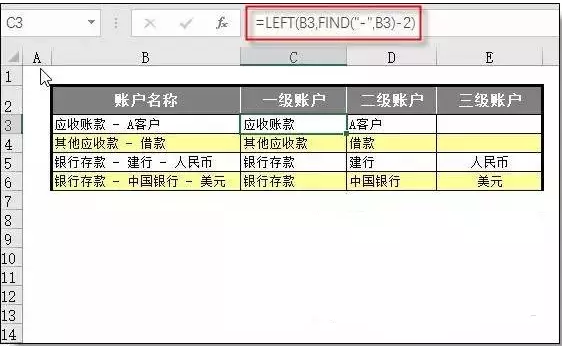19、多表同一位置汇总公式

B2 =SUM(Sheet1:Sheet19!B2)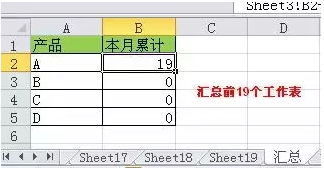20、中国式排名公式

=SUMPRODUCT((\$D\$4:\$D\$9>=D4)*(1/COUNTIF(D\$4:D\$9,D\$4:D\$9)))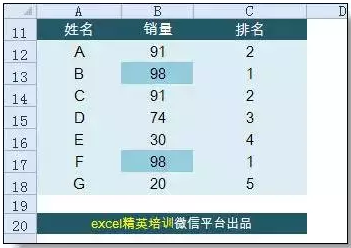21、先进先出公式

Lj

=SUBTOTAL(9,OFFSET(!\$E\$2,,,ROW()-1))-SUBTOTAL(9,OFFSET(!\$B\$2,,,ROW(!\$B\$1:\$B9)))

G3公式：

{=SUMPRODUCT(IF(lj>\$B\$3:B3,\$B\$3:B3,lj),IF(lj>0,\$C\$3:C3))-SUM(\$G\$2:G2)}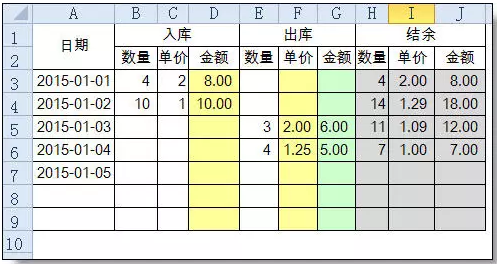## 相关问答

• 【答】你好！如是去加油站加油取得发票入账是不需要分别核算。苏颖莹

咨询老师
• 【答】你好，你的问题过于宽泛，请你参考真账实训里的医院和民办学校及幼儿园，如果没有适合你们单...张张老师

咨询老师
• 【答】你好，你看下出售时候的会计分录，公允价值变动损益是把金额转给投资收益了，，计算净损益，...张张老师

咨询老师